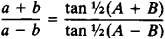# Tangents, Law of

The following article is from The Great Soviet Encyclopedia (1979). It might be outdated or ideologically biased.

## Tangents, Law of

a trigonometric theorem giving a relation between the lengths of two sides of a triangle and the tangents of half the sum and half the differences of the opposite angles. The law can be stated as follows:where a and b are the lengths of two sides of a triangle and A and B are the opposite angles. This particular form of the law is due to Vieta.